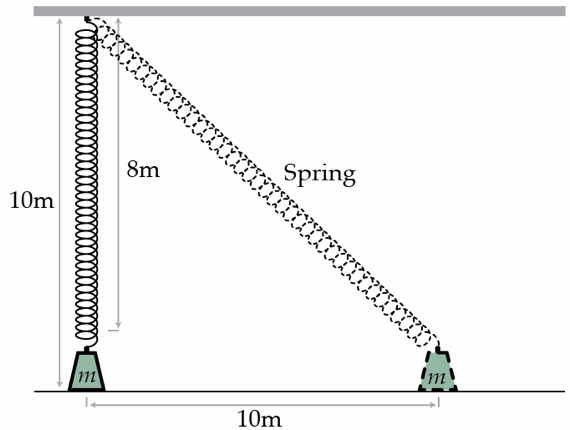# Spring to the top

A heavy mass is attached to a spring of natural length $8~\mbox{m}$ and spring constant $k=100~\mbox{N/m}$. The top of the spring is attached to a ceiling, which is $10~\mbox{m}$ above the mass. The mass is then pulled horizontally for a distance of $10~\mbox{m}$ and released. It therefore slides back along the ground towards where it started. What is the kinetic energy of the mass in Joules when it returns to its starting point?Details and assumptions

• The floor is frictionless.
• The mass is heavy enough that it always rests on the ground.
• Treat the mass as a point mass.
×